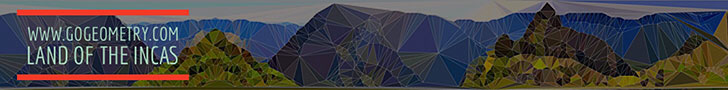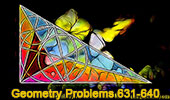Geometry Problems 631-640: Triangle, Collinearity, Incenter, Circle, Arbelos# Geometry Problems 631 - 640Geometry Problem 631.
Triangle, Exterior Angle Bisectors, Collinear Points.

Geometry Problem 632.
Triangle, Interior and Exterior Angle Bisectors, Midpoints, Collinear Points.

Geometry Problem 633
Triangle, Parallel Lines, Intersecting Lines.

Geometry Problem 634
Right Triangle, Altitude, Incenters, Areas.
Geometric art of problem 634.
Background: stereographic projection of the Temple of the Three Windows at Machu Picchu

Geometry Problem 635
Semicircle, Diameter, Perpendicular, Inscribed Circle, Radius.

Geometry Problem 636
Semicircle, Diameter, Perpendicular, Inscribed Circle, Common Tangent.

Geometry Problem 637
Semicircle, Diameter, Perpendicular, Inscribed Circle, Chord, Tangent, Arbelos.

Geometry Problem 638
Arbelos and Inscribed Circle: Concyclic Points.

Geometry Problem 639
Semicircle, Diameter, Perpendicular, Inscribed Circle, Tangent, Arbelos, Congruence.

Geometry Problem 640.
Archimedes Book of Lemmas: Proposition 1
Tangent circles and parallel diameters

Etching and Typography of Geometry Problem 640.
Archimedes Book of Lemmas: Proposition 1